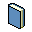Vista normal Vista MARC Vista ISBD

# Modeling and simulation of chemical process systems / Nayef Mohamed Ghasem.

Tipo de material:LibroEditor: Florida : CRC press, 2019Descripción: 502 p. : 25 cm.ISBN: 9781138568518 .Resumen: The textbook teaches readers how to model and simulate a unit process operation through developing mathematical model equations, solving model equations manually, and comparing results with efficient software. The book covers both lumped parameter systems and distributed parameter systems and uses MATLAB and Simulink to solve the system model equations for lumped parameter systems and for distributed parameter systems generated simplified partial differential equations are solved using COMSOL, an effective tool to solve PDE using fine element method. The book includes end of chapter problems and worked examples and summarizes reader goals at start of every chapter-- Provided by publisher.
valoración media: 0.0 (0 votos)
Tipo de ítem Ubicación actual Colección Signatura Copia número Estado Fecha de vencimiento Código de barrasLibro Biblioteca Central Mexicali
Acervo General TP184 .G483 2018 (Navegar estantería) 1 Disponible MXL122095
##### Navegando Biblioteca Central Mexicali Estantes , Código de colección: Acervo General Cerrar el navegador de estanterías
 TP156 .F6 F5818 1992 Flujo de fluidos en válvulas, accesorios y tuberías / TP156.P6 E53 2014 Engineering of polymers and chemical complexity : TP159 V34 1992 Válvulas : TP184 .G483 2018 Modeling and simulation of chemical process systems / TP248.16 D68 2013 Downstream industrial biotechnology : TP248.2 C4918 2012 Biotecnología en 26 fichas / TP248.2 I35 2012 Biotechnology :

incluye referencias bibliográficas

The textbook teaches readers how to model and simulate a unit process operation through developing mathematical model equations, solving model equations manually, and comparing results with efficient software. The book covers both lumped parameter systems and distributed parameter systems and uses MATLAB and Simulink to solve the system model equations for lumped parameter systems and for distributed parameter systems generated simplified partial differential equations are solved using COMSOL, an effective tool to solve PDE using fine element method. The book includes end of chapter problems and worked examples and summarizes reader goals at start of every chapter-- Provided by publisher.Con tecnología Koha

// {lang: 'es-ES'} //